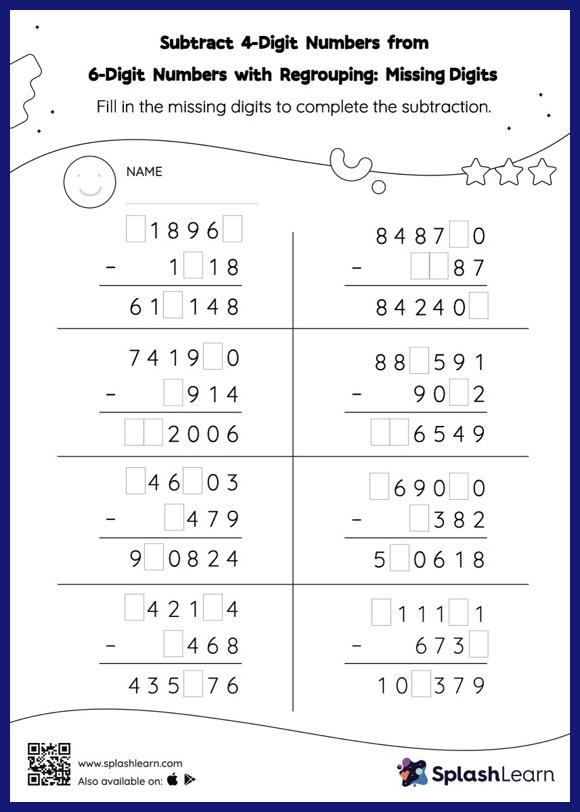# Subtract 4-Digit Numbers from 6-Digit Numbers with Regrouping: Missing Digits Worksheet

Home > Subtract 4-Digit Numbers from 6-Digit Numbers with Regrouping: Missing DigitsThis worksheet challenges young mathematicians to hone their math skills by solving a set of problems on subtracting 4-digit numbers from 6-digit numbers with regrouping. To find the missing number on this worksheet, students can utilize the count back strategy or the connection between addition and subtraction. In subtract 4-digit numbers from 6-digit numbers with regrouping worksheet, they must additionally regroup numbers to reach the solution.